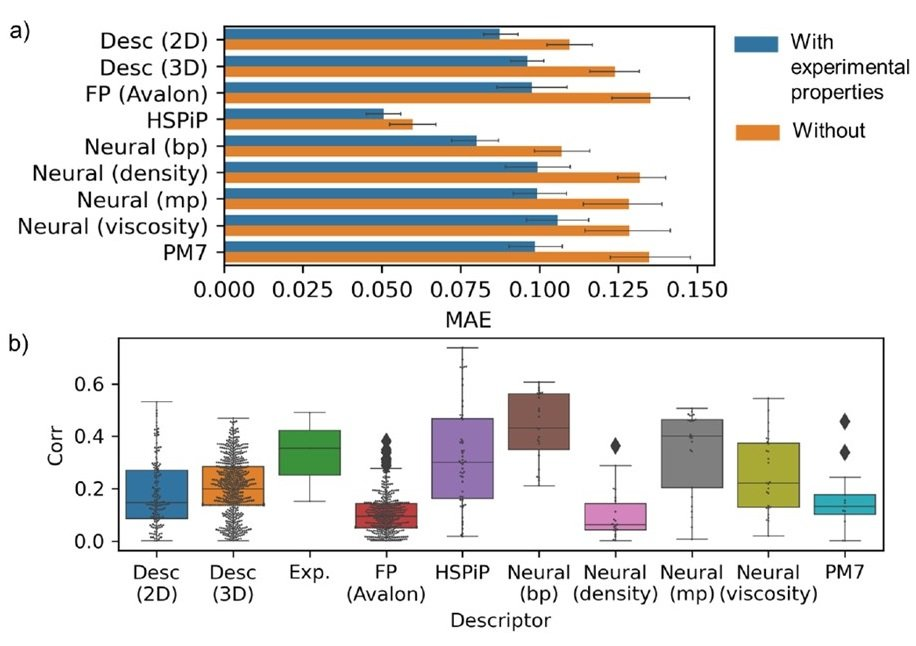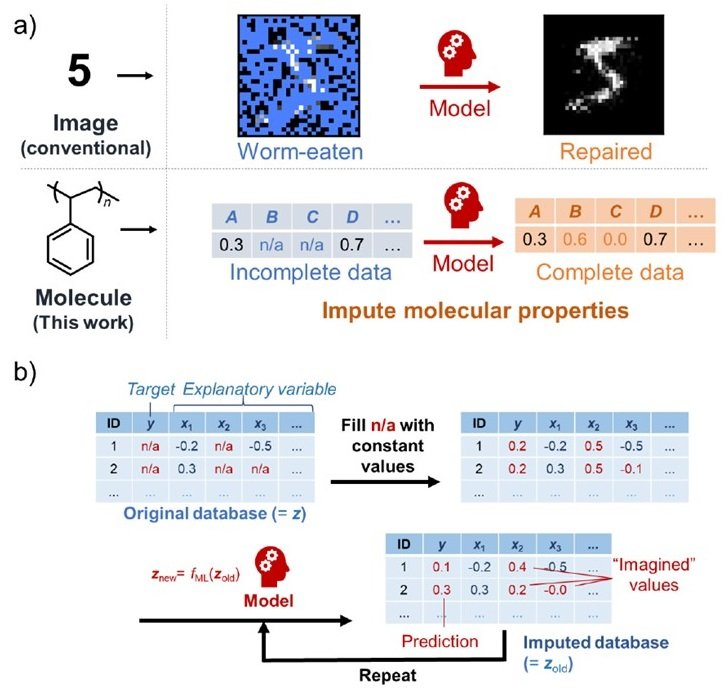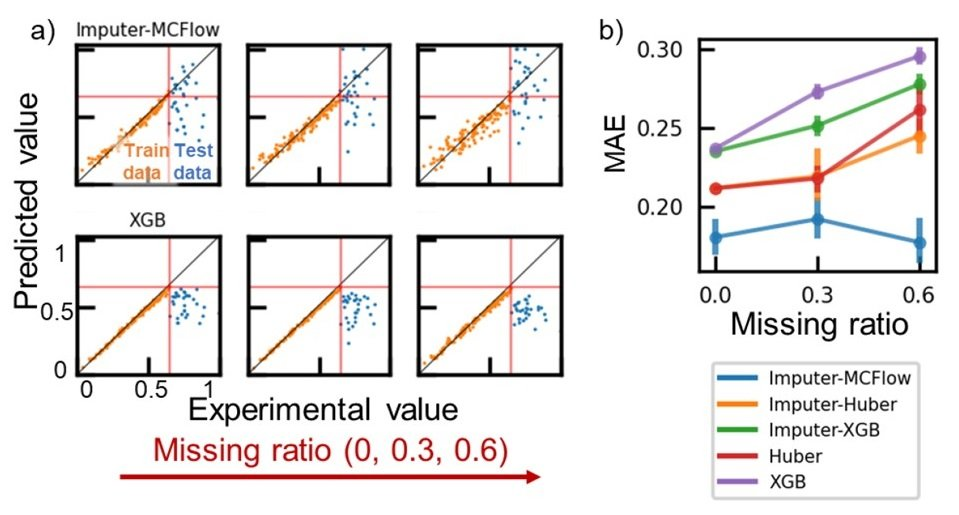# Catch up on the latest AI articles# A Generative Model For Extrapolation Tasks That Is Robust To Missing Data [Materials Informatics].

3 main points
✔️ Improve prediction accuracy by "imagining" and complementing missing data
✔️ Enables extrapolation predictions that are critical for unknown substance discovery using a small database
✔️ Improves accuracy by more than 30% over linear regression, the only practical extrapolation model

The images used in this article are from the paper or created based on it.

## introduction

Prediction by MI is promising because it is more accurate in some cases than conventional theory-based prediction and computational simulation. In particular, MI is more suitable in many cases for theoretical calculations of complex systems because of the explosive increase in cost.

However, in many cases, conventional MI methods predict only one physical property from limited information of a limited type of material. Therefore, it is desirable to develop a more flexible and human-like algorithm that can acquire and utilize a wide range of knowledge about materials as desired.

In many cases, the amount of data (information) available for MI is limited. As a countermeasure, transfer learning is a powerful approach, but it can be difficult to collect suitable data to use for pre-training. The data available from laboratory experiments is ~104 at most. The data obtained in computational science is inherently different from the data obtained in experiments, and computational science uses many approximations, so it is not easy to obtain data that "exactly reproduces" the phenomenon. (It can be done to some extent if you don't care about those problems in detail...)

Therefore, in the current situation, there is a high demand for approaches that can make robust predictions with small amounts of data.

In the generative model proposed in this study, we do not only look at the relationship between a particular explanatory variable x and the target variable y, but also a wide range of data inputs. It then uses a small and incomplete database to generate additional data for training by "imagining" to predict various properties.

Furthermore, in this study, we go into the most important "extrapolation task" in the search for unknowns in MI. The extrapolation task is difficult, and except for linear regression, there are few practical methods in the field of MI that are robust to "extrapolation".

## descriptor selection

Molecular descriptors, fingerprints, neural network outputs, and structure-related properties (e.g., orbital energies of molecules) are used as descriptors for organic molecules. However, since each descriptor has been developed independently, there is no clear guideline for descriptor selection. In this study, we first screened descriptors in a database of organic molecules (a small database of 160 types of small molecules including H, C, N, O, S, P, and halogens).

Boiling point, melting point, density, and viscosity were recorded as experimental values. These physical property values were learned and predicted using the following descriptor algorithm.

• Two- or three-dimensional geometric molecular descriptors (Desc 2D or 3D)
• fingerprints (FPs)
• Descriptors considering experimental values (HSPiP)
• neural descriptors
• Properties of single molecules calculated by semiempirical molecular orbital methods such as PM7

The neural descriptors are pre-learned by predicting the above four parameters from the molecular structure represented by the graph structure. XGB (Extreme Gradient Boosting) was used as a model, and each descriptor was used to learn the physical properties of the target molecule. Incidentally, in this training, we also used three other experimental values besides the target variable as explanatory variables ((e.g.) To predict the boiling point, we use melting point, density, and viscosity).

The prediction error for each descriptor resulting from the prediction of the boiling points of organic molecules is shown in (a) below.(b) shows a comparison of the correlations between the explanatory variables (the results of the molecules described by the descriptors) and the objective variable (the boiling point).

HSPiP descriptor is the best performance and The pre-trained Neural descriptor also performed well. In addition, when we added not only descriptor but also experimental parameters such as melting point (blue bar) in the explanatory variable x, the performance was remarkably better than the descriptor alone. In addition, the with neural descriptors. trained for the melting point also performed well in predicting the boiling point. This may be due to the strong correlation between the two parameters. However, the in neural descriptors. However, the transfer learning in neural descriptors did not always give good results. This is because of the physical property parameters' wide variety and the small size of our database.

Also, the purpose is to change the physical properties from boiling point to melting point, viscosity, density, etc. the effective descriptor also changes. Therefore, it can be said that no descriptor is effective for all.

However, when another experimental value was added to x, the performance improved, which means that the experimental value is a good descriptor. Furthermore, another test shows that a strong linear correlation between the explanatory and target variables improves the performance (Figure (b) above). Therefore, it can be said that a strong correlation between explanatory and objective variables is necessary for machine learning on a small database like this one.

## Model building and evaluation

Experimental values are valid explanatory variables but compared to general descriptor use or simulation. measurement costs. For this reason, databases of experimental values are very often full of holes.

In this study, we propose a generative model that has the feature of being robust to holey databases. Taking image repair as an example, this generative model can predict the original image by "imagining" the missing data part of the bug-eaten image. This is made possible by considering the relationships between the observed variables, just as humans do. In this article, we will apply this technique to material databases. The assignment process by "imagining" the missing values goes as follows.

1. First, the holey parts of x and y in the database are replaced by constants ( z old ) in the database.
2. Next, we train the proposed model ( z new = fML ( z old )) along with an imitation record of the data variance.
3. The predictions from the obtained model ( z new ) with the first constant ( z old ) are replaced by
4. Repeat this until convergence is achieved.

This sequence of processes makes the perforated database complete.As in the case of descriptor selection, we predicted four physical properties from the structural information. Here, we used Desc 2D (a descriptor of 2D geometric molecular structure) as a descriptor. Then, to reproduce the whole formation, we created a database in which the data were randomly deleted at 0, 30, and 60%. The data were To test the extrapolation task, we used the objective We used the top 20% of the variables with the highest values as test data, and The rest was used as training data.

As a generative model, we used a flow-based model called "Monte Carlo Flow (MCFlow)" as an assigner. This framework achieves state-of-the-art performance on a sequence of assignment tasks, including image repair. Appropriate randomness and reversible mapping functions are said to play an important role in prediction. Compared to other deep generative models such as autoencoders and GANs, this framework is capable of more accurate assignments in small databases, which is why we used it in this work.

With this generative model extrapolation prediction of the boiling point on a data set with a data loss ratio of 0.6, the MAE was smaller than 0.2, whereas the MAE for XGB was about 0.3. This may be because XGB is not suitable for extrapolation. Most decision-tree-type regression models, including random forests and ensemble models, have algorithms that are specialized for unique classifications. Therefore, they are not suitable for extrapolation tasks, and the results are generally similar to those of the XGB.Imputer-XGB: Result of prediction by XGB model with missing values filled in somehow such as mean value

The only practical application in the field of extrapolation is linear regression. The loss data are replaced by the mean, and a linear regression model is constructed using the Huber loss function to make a similar prediction was performed. As a result, the MAE was 0.20 to 0.25, which is better than XGB but not as good as MCFlow. Thus, the MCFlow model may be an excellent extrapolation model to replace linear regression.

The generative model first uses the mapping function f map to transform the original input data z into a vector z map of the same dimension. The z map is then converted to ^zmap by a multilayer perceptron for maximum density estimation. Then reconstruct z with the inverse function f map-1.

( *f ma is designed to be a reversible function.)

Interestingly, the explanatory (latent) variables tend to be more strongly correlated with the objective variable after the above transformation. This ability to extract the "underlying linearity between explanatory and target variables" is thought to contribute strongly to the achievement of high precision extrapolation.

We performed the same prediction task with GAN, autoencoder (AE), and variational autoencoder. The results showed that GAN failed to learn. This is because GAN failed to learn, probably because it had only about 150 training data despite deep learning. AE and VAE were not badly accurate, but they were not predictable in the extrapolation region.

This difference in performance between the flow-based model and the autoencoder can be attributed to the difference in whether the inverse transformation is possible or not.

MissForest, a random forest assigner, gave better extrapolation prediction performance than other popular models even with small databases. This framework is a derivative of the generative model because it estimates the distribution of z from the input data.

As described above, MCFlow has shown excellent extrapolation performance, but we believe that even better extrapolation and interpolation prediction performance can be obtained by optimizing the hyperparameters and net structure.

## Predictive performance of various physical properties

To investigate the performance of the generative model, several databases were used to predict the physical properties. The database we used covers 12000 compounds and more than 25 physical properties such as glass transition temperature, specific heat, viscosity, vapor pressure, etc., including many missing values. Using this database, the missing values were substituted and predicted by the present generative model. We used 2D molecular descriptor as a descriptor and predicted various physical properties by adding physical properties other than the target variable and repeating the regression task. The top 20% of the target variables were used to evaluate the extrapolation task, and the randomly selected 20% were used to evaluate the interpolation task.

As an example, we predicted the viscosity of an organic molecule based on about 100 data sets and found that MCFlow improved the value of the loss function by nearly 30% over Huber (a linear regression model built using the Huber loss function) in extrapolation, and similar results were obtained in interpolation. Similar results were obtained in interpolation.

In addition, the evaluation was performed using the reproduction rate and the fit rate defined as follows as evaluation indices.

Recall = Percentage of accurate predictions in the extrapolation region

Precision = Percentage of candidates with good interpolation performance that are not found by mistake.

As a result, Huber obtained 0% in both cases, while MCFlow obtained 19% and 75%, respectively (see b) above). Considering the difficulty of the extrapolation task, the MCFlow value of 19% is a good first step.

When the number of data used was less than 100, MCFlow was superior, but when the number of data used exceeded 100, the prediction accuracy of MCFlow and Huber became similar. However, when the number of data exceeds 100, the prediction accuracy of MCFlow and Huber becomes comparable. This may be because each predictor is given enough information when the number of data exceeds 100. However, MCFlow is a superior model because it can make accurate predictions with a smaller amount of data since it is costly and time-consuming to create a database through experiments.

## Comparison with other models

Linear regression, such as Huber, is a simple and robust model, but it has limitations in its use. This is because complex chemical and physical phenomena consisting of many different elements are not always amenable to linear regression.

Transfer learning cannot be applied unless the amount of data required for pre-training is sufficient. Models using graph structures as descriptors are powerful methods, but again, they cannot be applied when the amount of data is small. In addition, the predictions of transition learning and graph-structured models are black-boxed, which makes it difficult for researchers to understand the correlation between the structure and properties of materials. On the other hand. On the other hand, the generative model proposed here has an advantage over both of them. On the other hand, the proposed generative model has an advantage over both of them: the correlation between the structure and the physical properties is explicitly expressed as a "generated database".

Furthermore, flow-based generative models excel at extrapolation tasks. On the other hand, algorithms used in other MIs are often specialized for interpolation tasks.

## summary

In this paper, we proposed a generative model that can substitute and predict a variety of information about different materials. By "imagining" the missing data, we were able to improve the prediction accuracy in interpolation and extrapolation tasks. This can be said to be close to what we humans do. In particular, the extrapolation task is the most important in the search for unknown substances, and we hope that the results of this research will lead to breakthroughs in MI.

If you have any suggestions for improvement of the content of the article,